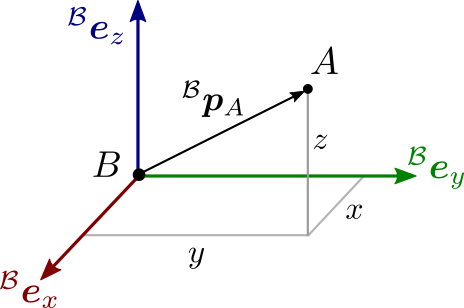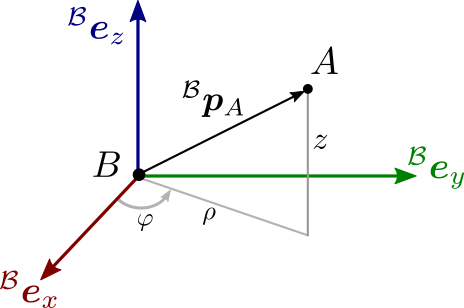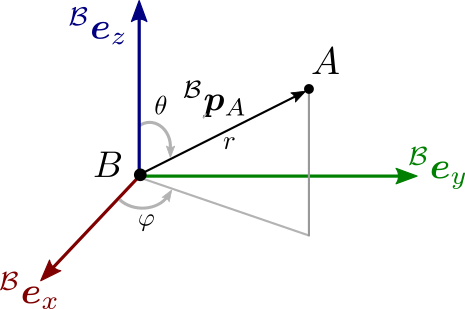# Position and coordinate systems

The simplest object we can study in kinematics is the point, also known as particle in classical mechanics. We can describe a point $$A$$ in the Euclidean space by providing a vector $${}^\calB \bfp_A \in \mathbb{R}^3$$ of coordinates in the reference frame $$\calB = (B, {}^\calB \bfe_x, {}^\calB \bfe_y, {}^\calB \bfe_z)$$.

## Cartesian coordinates

The three vectors $$({}^\calB \bfe_x, {}^\calB \bfe_y, {}^\calB \bfe_z)$$ form an orthonormal basis of $$\mathbb{R}^3$$. We can use it to express the Cartesian coordinates of $$A$$, which consist of:

• The signed distance $$x$$ along the x-axis.
• The signed distance $$y$$ along the y-axis.
• The signed distance $$z$$ along the z-axis.

Mathematically:

\begin{equation*} {}^\calB \bfp_A = \begin{bmatrix} x \\ y \\ z \end{bmatrix} = x\,{}^\calB \bfe_x + y\,{}^\calB \bfe_y + z\,{}^\calB \bfe_z \end{equation*}

Graphically:On a side note, the figure above introduces a color convention from computer graphics where the x-axis (sagittal) is red, the y-axis (lateral) green and the z-axis (vertical) blue. This convention is common in off-the-shelf robotics software such as RViz and OpenRAVE.

## Cylindrical coordinates

Cylindrical coordinates represent the position of a point relative to an axis of interest, typically the vertical z-axis, and a direction of interest, typically the sagittal x-axis. They consist of three numbers:

• The distance $$\rho$$ from $$A$$ to the z-axis.
• The angle $$\varphi$$, called azimuthal angle (or just the azimuth, which sounds way cooler), between the vector from $$B$$ to $$A$$ and $$\bfe_x$$.
• The axis coordinate $$z$$.

Mathematically, we can map the vector $${}^\cal B\bfchi_A$$ of cylindrical coordinates to Cartesian ones by:

\begin{equation*} {}^\calB \bfchi_A = \begin{bmatrix} \rho \\ \varphi \\ z \end{bmatrix} \ \Longrightarrow \ {}^\calB \bfp_A({}^\calB \bfchi_A) = \begin{bmatrix} \rho \cos \varphi \\ \rho \sin \varphi \\ z \end{bmatrix} \end{equation*}

Graphically:These coordinates are sometimes useful to analyze quantities that act on a body frame of a car or mobile robot. For example, when an external disturbance is applied to a humanoid robot, looking at the direction and amplitude of the disturbance in the horizontal plane can be more insightful than looking at individual x- and y-coordinates.

## Spherical coordinates

Spherical coordinates represent the position of a point by:

• Its distance $$r$$ to the origin $$B$$ of the reference frame.
• The angle $$\varphi$$, the azimuth™, identical to the one used in cylindrical coordinates.
• The angle $$\theta$$, called polar, corresponding to the amount of rotation to apply in the resulting plane to go from the "pole" to the desired point.

Mathematically, we can map the vector $${}^\cal B\bfsigma_A$$ of spherical coordinates to Cartesian ones by:

\begin{equation*} {}^\calB \bfsigma_A = \begin{bmatrix} r \\ \varphi \\ \theta \end{bmatrix} \ \Longrightarrow \ {}^\calB \bfp_A({}^\calB \bfsigma_A) = \begin{bmatrix} r \cos \varphi \sin \theta \\ r \sin \varphi \sin \theta \\ r \cos \theta \end{bmatrix} \end{equation*}

Graphically:## Observations

### Difference between the object and its coordinates

These three coordinate systems can be used equivalently to represent the position of our point $$A$$ in the reference frame $$\calB$$. Note how we have made, and will consistently make, the distinction between the point itself $$A$$ and the vector $${}^\calB \bfp_A$$ (of coordinates (with respect to a given reference frame)) used to represent this point mathematically. The same point can for instance be written $${}^\calC p_A$$ in another reference frame $$\calC$$ translated with respect to $$\calB$$. This mental gymnastics of keeping track of both the object and its representation will become all the more important as we move on to linear velocities, angular velocities, twists, etc.

### Omitting the reference frame

When there is no ambiguity, the reference frame exponent is often dropped and the position of point $$A$$ is abbreviated as $$\bfp_A$$. Yet, physical quantities are always expressed with respect to a reference frame, so implicitly there is always an underlying frame to any vector $$\bfp_A$$ of position coordinates.

Pages of this website are under the CC-BY 4.0 license.